Shepherd

The shepherd has fewer than 500 sheep; where they can be up to 2, 3, 4, 5, 6 row is always 1 remain, and as can be increased up to 7 rows of the sheep, and it is not increased no ovine. How many sheep has a shepherd?

Result

n =  301

Solution:

a%b = a modulo b

n=7: n%2=1, n%3=1, n%4=3, n%5=2, n%6=1, n%7=0
n=14: n%2=0, n%3=2, n%4=2, n%5=4, n%6=2, n%7=0
n=21: n%2=1, n%3=0, n%4=1, n%5=1, n%6=3, n%7=0
n=28: n%2=0, n%3=1, n%4=0, n%5=3, n%6=4, n%7=0
n=35: n%2=1, n%3=2, n%4=3, n%5=0, n%6=5, n%7=0
n=42: n%2=0, n%3=0, n%4=2, n%5=2, n%6=0, n%7=0
n=49: n%2=1, n%3=1, n%4=1, n%5=4, n%6=1, n%7=0
n=56: n%2=0, n%3=2, n%4=0, n%5=1, n%6=2, n%7=0
n=63: n%2=1, n%3=0, n%4=3, n%5=3, n%6=3, n%7=0
n=70: n%2=0, n%3=1, n%4=2, n%5=0, n%6=4, n%7=0
n=77: n%2=1, n%3=2, n%4=1, n%5=2, n%6=5, n%7=0
n=84: n%2=0, n%3=0, n%4=0, n%5=4, n%6=0, n%7=0
n=91: n%2=1, n%3=1, n%4=3, n%5=1, n%6=1, n%7=0
n=98: n%2=0, n%3=2, n%4=2, n%5=3, n%6=2, n%7=0
n=105: n%2=1, n%3=0, n%4=1, n%5=0, n%6=3, n%7=0
n=112: n%2=0, n%3=1, n%4=0, n%5=2, n%6=4, n%7=0
n=119: n%2=1, n%3=2, n%4=3, n%5=4, n%6=5, n%7=0
n=126: n%2=0, n%3=0, n%4=2, n%5=1, n%6=0, n%7=0
n=133: n%2=1, n%3=1, n%4=1, n%5=3, n%6=1, n%7=0
n=140: n%2=0, n%3=2, n%4=0, n%5=0, n%6=2, n%7=0
n=147: n%2=1, n%3=0, n%4=3, n%5=2, n%6=3, n%7=0
n=154: n%2=0, n%3=1, n%4=2, n%5=4, n%6=4, n%7=0
n=161: n%2=1, n%3=2, n%4=1, n%5=1, n%6=5, n%7=0
n=168: n%2=0, n%3=0, n%4=0, n%5=3, n%6=0, n%7=0
n=175: n%2=1, n%3=1, n%4=3, n%5=0, n%6=1, n%7=0
n=182: n%2=0, n%3=2, n%4=2, n%5=2, n%6=2, n%7=0
n=189: n%2=1, n%3=0, n%4=1, n%5=4, n%6=3, n%7=0
n=196: n%2=0, n%3=1, n%4=0, n%5=1, n%6=4, n%7=0
n=203: n%2=1, n%3=2, n%4=3, n%5=3, n%6=5, n%7=0
n=210: n%2=0, n%3=0, n%4=2, n%5=0, n%6=0, n%7=0
n=217: n%2=1, n%3=1, n%4=1, n%5=2, n%6=1, n%7=0
n=224: n%2=0, n%3=2, n%4=0, n%5=4, n%6=2, n%7=0
n=231: n%2=1, n%3=0, n%4=3, n%5=1, n%6=3, n%7=0
n=238: n%2=0, n%3=1, n%4=2, n%5=3, n%6=4, n%7=0
n=245: n%2=1, n%3=2, n%4=1, n%5=0, n%6=5, n%7=0
n=252: n%2=0, n%3=0, n%4=0, n%5=2, n%6=0, n%7=0
n=259: n%2=1, n%3=1, n%4=3, n%5=4, n%6=1, n%7=0
n=266: n%2=0, n%3=2, n%4=2, n%5=1, n%6=2, n%7=0
n=273: n%2=1, n%3=0, n%4=1, n%5=3, n%6=3, n%7=0
n=280: n%2=0, n%3=1, n%4=0, n%5=0, n%6=4, n%7=0
n=287: n%2=1, n%3=2, n%4=3, n%5=2, n%6=5, n%7=0
n=294: n%2=0, n%3=0, n%4=2, n%5=4, n%6=0, n%7=0
n=301: n%2=1, n%3=1, n%4=1, n%5=1, n%6=1, n%7=0 <<<<<<=====

Leave us a comment of example and its solution (i.e. if it is still somewhat unclear...):Be the first to comment!To solve this example are needed these knowledge from mathematics:

Do you want to calculate greatest common divisor two or more numbers?

Next similar examples:

1. Children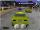Less than 20 children is played various games on the yard. They can create a pairs, triso and quartets. How many children were in the yard when Annie came to them?
2. TissuesThe store got three kinds of tissues - 132 children, 156 women and 204 men. Tissues each species were packed into boxes after the number of pieces the same for all three types (and greatest). Determine the number, if you know that every box has more than 6
3. Farm2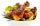The farm bred turkeys, geese, hens and chickens. Everything is 400. Turkeys and geese a hen are 150. No hen has more than one chicken, but some hens has no chick. Half of them is also a quarter of the chickens. When the turkey subtracting 15, we get 75. Ho
4. Pages of book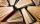Lenka calculated that if every day to read 16 pages of the book, read a book a day earlier than had read only 14 pages a day. How many pages has this book?
5. Donuts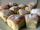In the dining room at one table sit Thomas, Filip and Martin. Together they eat 36 donuts. How many donuts eaten everyone, when Filip ate twice as much as Thomas and Martin even half over Filip?
6. Ski tow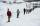The ski club has 168 pupils and used lift with 60 seats, while students always follow the same sequence in filling seats. How many times while riding a ski lift skier sitting in the same seat as the first run?
7. Toy carsPavel has a collection of toy cars. He wanted to regroup them. But in the division of three, four, six, and eight, he was always one left. Only when he formed groups of seven, he divided everyone. How many toy cars have in the collection?
8. RemainderA is an arbitrary integer that gives remainder 1 in the division with 6. B is an arbitrary integer that gives remainder 2 the division by. What makes remainder in division by 3 product of numbers A x B ?
9. Apples 2How many apples were originally on the tree, if the first day fell one third, the second day quarter of the rest and on tree remained 45 apples?
10. Bundle of candies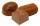In the store has 168 chocolates, caramel candies 224 and 196 hard candies. How many packages we can do and how many of candies will be in each package?
11. Divisible by 5How many three-digit odd numbers divisible by 5, which are in place ten's number 3?
12. DivisibilityWrite all the integers x divisible by seven and eight at the same time for which the following applies: 100
13. Divisibility by 12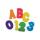Replace the letters A and B by digits so that the resulting number x is divisible by twelve /find all options/. x = 2A3B How many are the overall solutions?
14. DivisorsThe sum of all divisors unknown odd number is 2112. Determine sum of all divisors of number which is twice of unknown numbers.
15. Bicycle wheels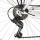Driving wheel of a bicycle has 54 teeth. The driven wheel has 22 teeth. After how many revolutions will meet the same teeth?
16. Football league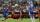In the 2nd football league plays each of the 16 participants with each opponent twice. For every victory gets 3 points, 1 point for a draw, a defeat none. After the competition was team with 54 points and 12 defeats at 9th place. How many times have won?
17. Buttons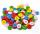Shirt has 6 buttons, blouse has 4 buttons. All buttons are 176. Shirts and blouses are together 36. How many are the shirts and blouses?